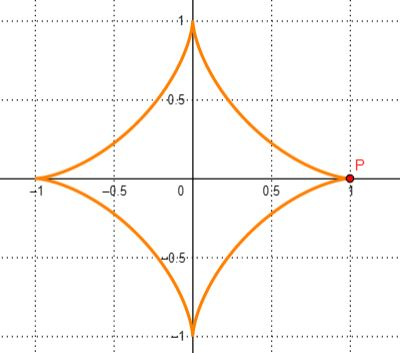# The curve that is parametrized by x(t) = \cos^3 t and y(t) = \sin^3 t, with 0 \leq t \leq 2\pi,...

## Question:

The curve that is parametrized by {eq}x(t) = \cos^3 t\text{ and }y(t) = \sin^3 t {/eq}, with {eq}0 \leq t \leq 2\pi {/eq}, is called a hypocycloid.

a. Show that the curvature is {eq}k(t) = \frac 1{|3\sin t \cos t|} {/eq} .

b. What is the minimum curvature and where on the curve does it occur?

c. For what t is the curvature undefined? At what points on the hypocycloid does that occur? Explain what is happening on the path at those point.

## Curvature of parametric functions

This example illustrates how to find the curvature of a function whose equation is specified parametrically.

The example goes on to investigate anomalous points on the graph of one such curve.

Part a

We will use the formula below for curvature:

{eq}\kappa = \dfrac{|x'y'' - y'x''|}{(x'^2 + y'^2)^{\frac{3}{2}}} {/eq}

and where the dashes are used to denote differentiation with respect to the parameter t.

Differentiating using the chain rule:

{eq}x(t) = \cos^3t \\ \Rightarrow x' = 3(\cos^2t)(-\sin t) = -3\cos^2t\sin t {/eq}

Differentiating again using a mixture of the product rule and chain rule:

{eq}x'' = (-3\cos^2t)(\cos t) + (-6\cos t(-\sin t))(\sin t) = -3\cos^3t + 6\sin^2t\cos t {/eq}

In a similar way:

{eq}y(t) = \sin^3 t \\ \Rightarrow y' = 3\sin^2t \cos t \\ \Rightarrow y'' = 3\sin^2t(-\sin t) + (6\sin t(\cos t))(\cos t) = -3\sin^3t + 6\sin t\cos^2t {/eq}

Then we have the curvature as:

{eq}\kappa = \dfrac{|x'y'' - y'x''|}{(x'^2 + y'^2)^{\frac{3}{2}}} \\ \Rightarrow \kappa = \dfrac{|(-3\cos^2t\sin t)(-3\sin^3t + 6\sin t\cos^2t) - (3\sin^2t \cos t)(-3\cos^3t + 6\sin^2t\cos t)|}{((-3\cos^2t\sin t)^2 + (3\sin^2t \cos t)^2)^{\frac{3}{2}}} \\ = \dfrac{|9\sin^4t\cos^2t - 18\sin^2t\cos^4t + 9\sin^2t\cos^4t - 18\sin^4t\cos^2t|}{(9\sin^2t\cos^4t + 9\sin^4t\cos^2t)^\frac{3}{2}} \\ = \dfrac{|-9\sin^4t\cos^2t - 9\sin^2t\cos^4t|}{(9\sin^2t\cos^2t(\cos^2t + \sin^2t))^\frac{3}{2}} \\ = \dfrac{9\sin^2t\cos^2t(\sin^2t + \cos^2t)}{(9\sin^2t\cos^2t(\cos^2t + \sin^2t))^\frac{3}{2}} \\ = \dfrac{9\sin^2t\cos^2t(1)}{(9\sin^2t\cos^2t(1))^\frac{3}{2}} \\ = \dfrac{9\sin^2t\cos^2t}{|27\sin^3t\cos^3t|} \\ = \dfrac{1}{|3\sin t\cos t|} {/eq}

as required.

Note the introduction of the modulus sign to ensure that the denominator maintains its positive state following the cancellation of terms.

Part b

Using the identity {eq}\sin t\cos t = \dfrac{1}{2}\sin 2t, {/eq} we have

{eq}\kappa = \dfrac{1}{|\dfrac{3}{2}\sin 2t|} = \dfrac{2}{3|\sin 2t|}. {/eq}

The minimum value of {eq}\kappa {/eq} will occur where the denominator is a maximum.

This occurs when {eq}\sin 2t = \pm1, {/eq} giving a minimum curvature {eq}\kappa = \dfrac{2}{3}. {/eq}

The corresponding value of t comes from

{eq}\sin 2t = \pm 1 \\ \Rightarrow 2t = \sin^{-1}(1) = \dfrac{1}{2}\pi \text { or } 2t = \sin^{-1}(-1) = \dfrac{3}{2}\pi \\ \Rightarrow t = \dfrac{1}{4}\pi \text { or } t = \dfrac{3}{4}\pi {/eq}

These values of t give

{eq}x = \cos^3(\dfrac{1}{4}\pi) = 0.35, \: y = \sin^3(\dfrac{1}{4}\pi) = 0.35 \\ x = \cos^3(\dfrac{3}{4}\pi) = -0.35, \: y = \sin^3(\dfrac{3}{4}\pi) = 0.35 {/eq}

i.e. the points {eq}(0.35, 0.35), \: (-0.35, 0.35). {/eq}

Part c

The curvature is undefined when the denominator of {eq}\kappa {/eq} is zero. Hence:

{eq}\sin 2t = 0 \\ \Rightarrow 2t = \sin^{-1}(0) = 0, \: \pi, \: \2\pi, \: 3\pi \\ \Rightarrow t = 0, \: \dfrac{1}{2}\pi, \: \pi, \dfrac{3}{2}\pi {/eq}

The corresponding x and y coordinates are easily found to be {eq}(1, 0), \: (0,1), (-1, 0), (0, -1). {/eq}

Below is a sketch of the curve.It can be seen that the 4 points found above are at the "cusps" of the curve, and as such the curve is not differentiable at hese points, hence the undefined curvature.